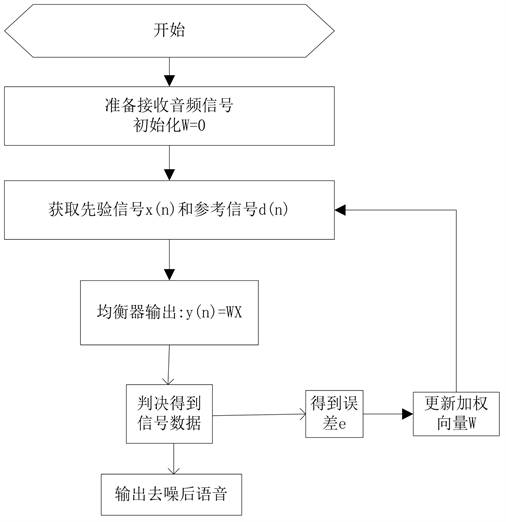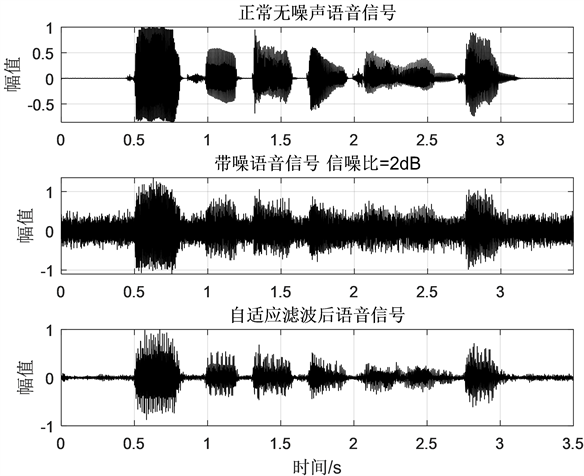﻿ 基于梯度下降的自适应音频去噪声的研究

Abstract: Filter skill deals with noise in background to compensate channel’s noise and reduce it. Commonly, we call the processor filter. The paper computes filter’s coefficient for minimum variance. The paper advises that one filter can reduce channel’s noise, base on gradient descent .For minimum variance between standard signal and noising signal, the filter’s coefficients will iterate to the last result. The simulation shows the filter can reduce noise to enhance SNR.

1. 引言

2. 自适应滤波数学理论

3. 迭代计算实现理论

$\eta =E\left\{x\right\}=\frac{1}{N}\underset{n=0}{\overset{N-1}{\sum }}x\left[n\right]$ (1)

$e\left[n\right]=d\left[n\right]-y\left[n\right]$ (2)

$j=E\left\{e{\left[n\right]}^{2}\right\}={\stackrel{¯}{\left(d\left[n\right]-y\left[n\right]\right)}}^{2}$ (3)

$e\left[n\right]=d\left[n\right]-y\left[n\right]=d\left[n\right]-{f}^{T}x\left[n\right]$ (4)

$J=E\left\{{e}^{2}\left[n\right]\right\}=E{\left\{d\left[n\right]-y\left[n\right]\right\}}^{2}=E{\left\{d\left[n\right]-{f}^{T}x\left[n\right]\right\}}^{2}$

$E\left\{d\left[n\right]-2d\left[n\right]{f}^{T}x\left[n\right]+{f}^{T}x\left[n\right]x\left[n\right]\right\}$ (5)

$\nabla =\frac{\partial J}{\partial {f}^{T}}=E\left\{-2d\left[n\right]x\left[n\right]+2x\left[n\right]x\left[n\right]{f}_{opt}\right\}=0$ (6)

${f}_{opt}=E\left\{x\left[n\right]{x}^{T}\left[n\right]\right\}E\left\{d\left[n\right]x\left[n\right]\right\}={R}_{xx}^{-1}{R}_{dx}$ (7)

LMS算法也是最速下降法的一种实现 ，根据梯度下降的方式推导得：

$f\left[n+1\right]=f\left[n\right]-\frac{\mu }{2}\nabla \left[n\right]$ (8)

$f\left[n+1\right]=f\left[n\right]+\mu e\left[n\right]x\left[n\right]$ (9)

4. 迭代计算自适应去噪滤波器系数的数学原理建模仿真实现Figure 1. Theory of adaptive filterFigure 2. Statistics the signal of audios

5. 迭代计算自适应去噪滤波器系数的数学原理建模仿真实现

6. 结论

 Douglas, S.C., Zhu, Q. and Smith, K.F. (1988) A Pipelined LMS Adaptive FIR Filter Architecture without Adaptation Delay. IEEE Transactions on Signal Processing, 46, 175-179.

 Simon Haykin 自适应滤波器原理[M]. 郑宝玉, 等, 译. 北京: 电子工业出版社, 2010.

 迈耶-贝斯. 数字信号处理的FPGA实现[M]. 陈青华, 等, 译. 北京: 清华大学出版社, 2017.

 卿朝进, 等. 一种基于绝对听觉阈值的深度学习语音增强方法[P]. CN109671446A 2019.10.12.

 赵力. 语音信号处理[M]. 北京: 机械工业出版社, 2019.

Top